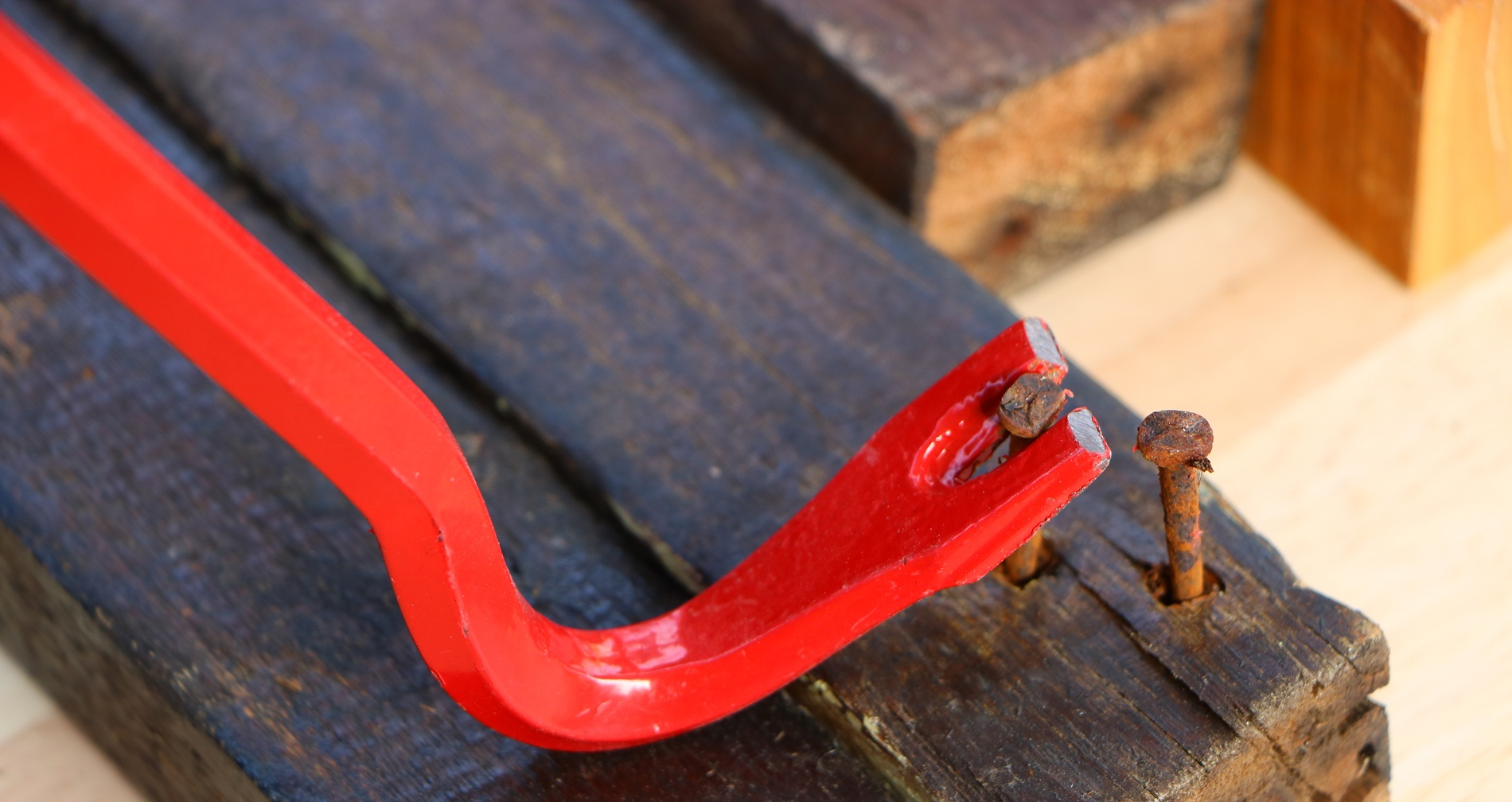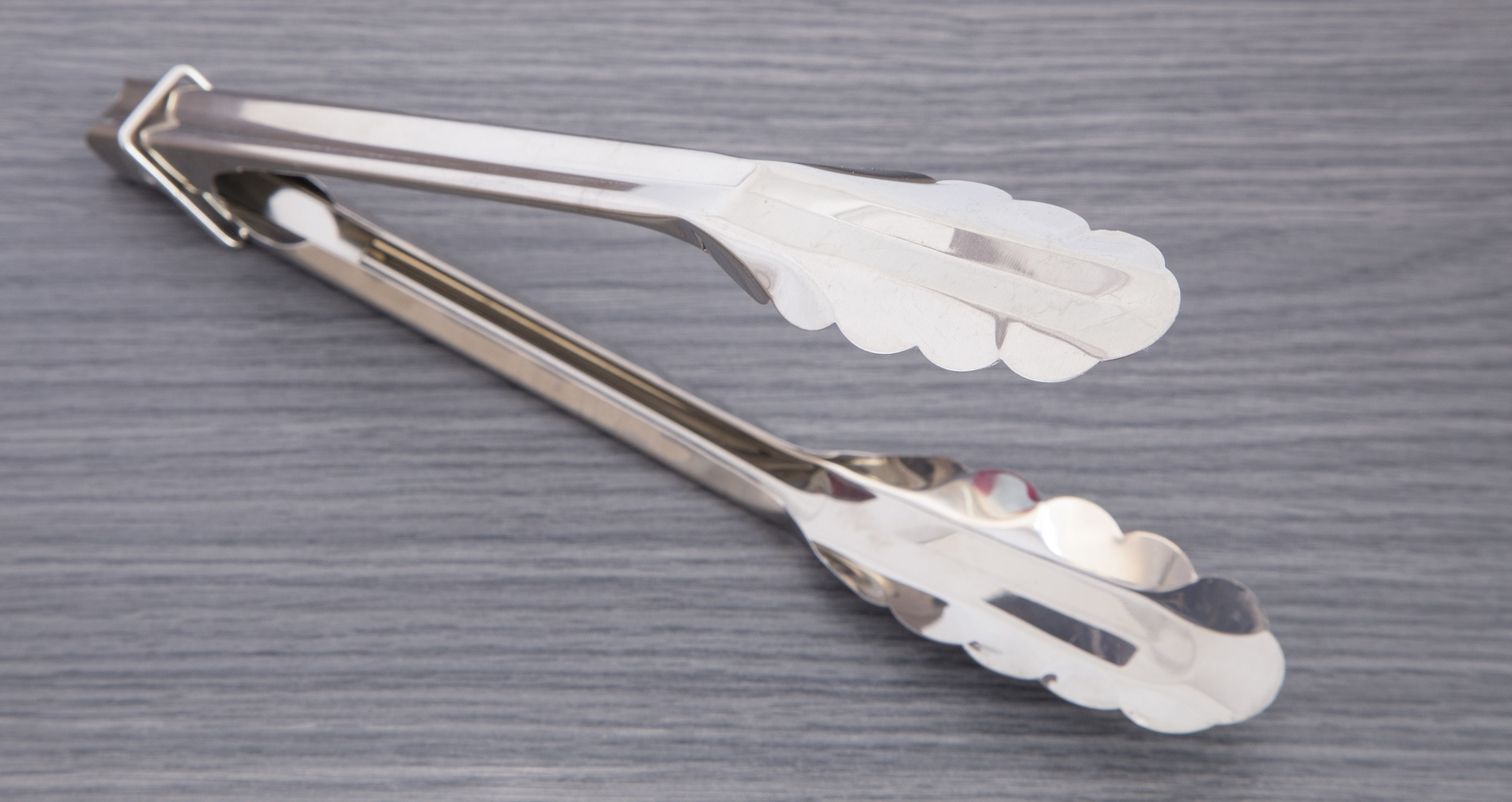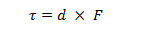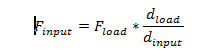# Lever Mechanisms

## What is a Lever?

Levers are mechanical devices used to scale the amount of force and/or travel in a mechanism.Levers are a versatile mechanism that can be used in a wide range of applications from small to large. For example, levers can be used to gain a mechanical advantage, allowing a small input force to exert a much higher output force. In other cases levers can be used to take a small amount of movement and multiply it into a larger movement. The most common use of levers is to create a mechanical advantage in order to assist in an action, as seen with pliers, door handles, pry bars, staplers, and wheelbarrows.## First Order Levers

First-order levers are mechanisms with the fulcrum placed between the input force and the output force. Think of a see-saw. In this case the input and output forces are equidistant from the fulcrum (meaning the output force will equal the input force less losses from friction).

Due to the input and output forces being on different sides of the fulcrum, the two forces will act in opposite directions. Crowbars and/or other prying tools are also great examples of first-order levers.

If the input force is further from the fulcrum than the output force (as in the case of a crowbar), the output force will be greater than the input force. However, the output travel will be less than the input travel. If the input force is closer to the fulcrum than the output force, the output force will be less than the input, but the output travel will be greater than the input travel. Another great example of this is a pair of garden sheers (see below):## Second Order Levers

Second-order levers are mechanisms which feature the input force and output force on the same side of the fulcrum, with the input force furthest from the fulcrum. A great example of a second-order lever is a wheelbarrow.

Similar to first-order levers, in a second-order lever the output force will be greater than the input force. However, the output travel will be less than the input travel. In this case, both the input and output forces will act in the same direction. In the wheelbarrow the output force in the tray sits between the input force on the handles and the fulcrum at the wheel axle. In the cases of first-order and second-order levers, the greatest mechanical advantage is achieved by placing the input force.## Third-order levers

Third-order levers also feature the input and output forces on the same side of the fulcrum, but in this case the output force is further from the fulcrum than the input force. As is the case with second-order levers, the input and output forces will act in the same direction. The benefit seen from third order levers is the larger travel distance of the output relative to the input. However, since the output is further from the fulcrum than the input, the output force will be less than the input force. Third order levers are the least common of the three types of levers. One example is kitchen tongs , where the hinge is the fulcrum, the input force comes from the user’s hands, and the output force is exerted at the tip of the tongs.

## Governing Equations For Levers

The same governing equation applies to all three orders of levers. When a force is applied somewhere on the lever, a torque is generated at the fulcrum. Torque is commonly given in units of lb-ft (pound feet) or N-m (Newton meters). The torque that each force generates is equal to the amount of force multiplied by the distance of that force from the fulcrum. Therefore, torque can be derived from the following equation:Where

τ is the torque,
d is the distance from the fulcrum, and
F is the force

By looking at the equation above, we can see that an increase in torque can be achieved by an increase in either distance or force. We can also see, in order to obtain a desired torque value, there are in infinite number of combinations of the force and distance values. A lot of force can be applied very close to the fulcrum or a very minimal force can be applied very far from the fulcrum. The force and distance will inversely change in order to maintain a desired torque value.

If the input force and distance are known, the torque about the fulcrum can be found as shown in the equation above. With that torque value, the same equation can be used to determine the output force if the output distance is known. Likewise, if the output force is known, the output distance can be found.

This relationship demonstrates that large loads can be lifted easily by a relatively smaller input force acting further away from the fulcrum. For example, if the load is 1ft away from the fulcrum while the input force is 2 feet away, the input force will only need to be half as much as the load to move the object. Generally speaking, the input force, or force required to move the load, can be expressed using the following equation:Where:

Finput is the input force,